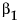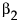Example of Equations of Fit
Summary of Fit Reports for Linear and Polynomial Fits
The RSquare values in Summary of Fit Reports for Linear and Polynomial Fits indicate that the polynomial fit of degree 2 gives a small improvement over the linear fit. See Summary of Fit Report.
Adjusts the RSquare value to make it more comparable over models with different numbers of parameters by using the degrees of freedom in its computation. See Summary of Fit Report.
Using the Lack of Fit report, you can estimate the error, regardless of whether you have the right form of the model. This occurs when multiple observations occur at the same x value. The error that you measure for these exact replicates is called pure error. This is the portion of the sample error that cannot be explained or predicted no matter what form of model is used. However, a lack of fit test might not be of much use if it has only a few degrees of freedom for it (few replicated x values).
Examples of Lack of Fit Reports for Linear and Polynomial Fits
The difference between the residual error from the model and the pure error is called the lack of fit error. The lack of fit error can be significantly greater than the pure error if you have the wrong functional form of the regressor. In that case, you should try a different type of model fit. The Lack of Fit report tests whether the lack of fit error is zero.
The three sources of variation: Lack of Fit, Pure Error, and Total Error.
The degrees of freedom (DF) for each source of error.
 – The Total Error DF is the degrees of freedom found on the Error line of the Analysis of Variance table (shown under the Analysis of Variance Report). It is the difference between the Total DF and the Model DF found in that table. The Error DF is partitioned into degrees of freedom for lack of fit and for pure error.
 – The Pure Error DF is pooled from each group where there are multiple rows with the same values for each effect. See Lack of Fit Report.
 – The Lack of Fit DF is the difference between the Total Error and Pure Error DF.
 – The Total Error SS is the sum of squares found on the Error line of the corresponding Analysis of Variance table, shown under Analysis of Variance Report.
 – The Pure Error SS is pooled from each group where there are multiple rows with the same value for the x variable. This estimates the portion of the true random error that is not explained by model x effect. See Lack of Fit Report.
 – The Lack of Fit SS is the difference between the Total Error and Pure Error sum of squares. If the lack of fit SS is large, the model might not be appropriate for the data. The F-ratio described below tests whether the variation due to lack of fit is small enough to be accepted as a negligible portion of the pure error.
The probability of obtaining a greater F-value by chance alone if the variation due to lack of fit variance and the pure error variance are the same. A high p value means that there is not a significant lack of fit.
The maximum R2 that can be achieved by a model using only the variables in the model. See Lack of Fit Report.
Analysis of variance (ANOVA) for a regression partitions the total variation of a sample into components. These components are used to compute an F-ratio that evaluates the effectiveness of the model. If the probability associated with the F-ratio is small, then the model is considered a better statistical fit for the data than the response mean alone.
The Analysis of Variance reports in Examples of Analysis of Variance Reports for Linear and Polynomial Fits compare a linear fit (Fit Line) and a second degree (Fit Polynomial). Both fits are statistically better from a horizontal line at the mean.
Examples of Analysis of Variance Reports for Linear and Polynomial Fits
The three sources of variation: Model, Error, and C. Total.
 – A degree of freedom is subtracted from the total number of non missing values (N) for each parameter estimate used in the computation. The computation of the total sample variation uses an estimate of the mean. Therefore, one degree of freedom is subtracted from the total, leaving 49. The total corrected degrees of freedom are partitioned into the Model and Error terms.
 – One degree of freedom from the total (shown on the Model line) is used to estimate a single regression parameter (the slope) for the linear fit. Two degrees of freedom are used to estimate the parameters (and) for a polynomial fit of degree 2.
 – The Error degrees of freedom is the difference between C. Total df and Model df.
 – In this example, the total (C. Total) sum of squared distances of each response from the sample mean is 57,278.157, as shown in Examples of Analysis of Variance Reports for Linear and Polynomial Fits. That is the sum of squares for the base model (or simple mean model) used for comparison with all other models.
 – For the linear regression, the sum of squared distances from each point to the line of fit reduces from 12,012.733. This is the residual or unexplained (Error) SS after fitting the model. The residual SS for a second degree polynomial fit is 6,906.997, accounting for slightly more variation than the linear fit. That is, the model accounts for more variation because the model SS are higher for the second degree polynomial than the linear fit. The C. total SS less the Error SS gives the sum of squares attributed to the model.
The sum of squares divided by its associated degrees of freedom. The F-ratio for a statistical test is the ratio of the following mean squares:
 – The Model mean square for the linear fit is 45,265.4. This value estimates the error variance, but only under the hypothesis that the model parameters are zero.
 – The Error mean square is 245.2. This value estimates the error variance.
The observed significance probability (p-value) of obtaining a greater F-value by chance alone if the specified model fits no better than the overall response mean. Observed significance probabilities of 0.05 or less are often considered evidence of a regression effect.
For a polynomial fit of order k, there is an estimate for the model intercept and a parameter estimate for each of the k powers of the X variable.
Examples of Parameter Estimates Reports for Linear and Polynomial Fits
Lists the observed significance probability calculated from each t-ratio. It is the probability of getting, by chance alone, a t-ratio greater (in absolute value) than the computed value, given a true null hypothesis. Often, a value below 0.05 (or sometimes 0.01) is interpreted as evidence that the parameter is significantly different from zero.
To reveal additional statistics, right-click in the report and select the Columns menu. Statistics not shown by default are as follows:
Related Information
 • Fit Line
 • Fitting Menus

Help created on 9/19/2017